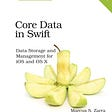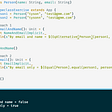# Scala: flatMap and for-comprehension

`object MyClass {    def twice(x: Int) = Some(x * 2)        def thrice(x: Int) = Some(x * 3)        def quadruple(x: Int) = Some(x * 4)def main(args: Array[String]) {        List(1,2,3)           .flatMap(twice)           .flatMap(thrice)           .flatMap(quadruple)           .foreach(println)           }244872`
`object MyClass {    def twice(x: Int) = Some(x * 2)        def thrice(x: Int) = Some(x * 3)        def quadruple(x: Int) = Some(x * 4)def main(args: Array[String]) {        List(1,2,3)           .flatMap(twice)           .flatMap(thrice)           .flatMap(quadruple)           .foreach(println)                 println("For comprehension")            (for {          n <- List(1,2,3)          t <- twice(n)          th <- thrice(t)          q  <- quadruple(th)      } yield q).foreach(println)    }}244872For comprehension244872`
`object MyClass {        case class Apple(s:String) {        override def toString() = s    }        def twice(x: Apple) = Some(Apple(x.toString + "-twice"))        def thrice(x: Apple) = Some(Apple(x.toString + "-thrice"))        def quadruple(x: Apple) = Some(Apple(x.toString + "-quadrupled"))def main(args: Array[String]) {        List(Apple("1"),Apple("2"),Apple("3"))           .flatMap(twice)           .flatMap(thrice)           .flatMap(quadruple)           .foreach(println)                 println("For comprehension")            (for {          n <- List(Apple("1"),Apple("2"),Apple("3"))          t <- twice(n)          th <- thrice(t)          q  <- quadruple(th)      } yield q).foreach(println)    }}1-twice-thrice-quadrupled2-twice-thrice-quadrupled3-twice-thrice-quadrupledFor comprehension1-twice-thrice-quadrupled2-twice-thrice-quadrupled3-twice-thrice-quadrupled`
`object MyClass {        case class Apple(s:String) {        override def toString() = s    }        case class Orange(s : String) {        override def toString() = s    }        def twice(x: Apple) = Some(Apple(x.toString + "-twice"))        def thrice(x: Apple) = Some(Apple(x.toString + "-thrice"))        def quadruple(x: Orange) = Some(Orange(x.toString + "-quadrupled"))def main(args: Array[String]) {        List(Apple("1"),Apple("2"),Apple("3"))          .flatMap(twice)          .flatMap(thrice)          .flatMap(quadruple)          .foreach(println)               }}jdoodle.scala:22: error: type mismatch; found   : Main.Orange => Some[Main.Orange] required: Main.Apple => scala.collection.IterableOnce[?]          .flatMap(quadruple)                   ^`
`object MyClass {        case class Apple(s:String) {        override def toString() = s    }        case class Orange(s : String) {        override def toString() = s    }        def twice(x: Apple) = Some(Apple(x.toString + "-twice"))        def thrice(x: Apple) = Some(Apple(x.toString + "-thrice"))        def quadruple(x: Orange) = Some(Orange(x.toString + "-quadrupled"))def main(args: Array[String]) {      println("For comprehension")            (for {          n <- List(Apple("1"),Apple("2"),Apple("3"))          t <- twice(n)          th <- thrice(t)          q  <- quadruple(th)      } yield q).foreach(println)    }}jdoodle.scala:25: error: type mismatch; found   : Main.Apple required: Main.Orange          q  <- quadruple(th)                          ^`

--

--

--

## More from Learn

On a continuing learning journey..

Love podcasts or audiobooks? Learn on the go with our new app.

## Day 76 | 100 Days Of GADS## Generic networking layer using Combine in Swift UI.## A Weather-Forecast App## Migrating an Existing Application## Learn

On a continuing learning journey..

## Scala: for-comprehension contexts do not mix## Polymorphic functions in Scala## Type Classes in Scala 2